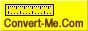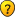# Linear feet around an acre.

Don't know how to calculate the area of your garden or circumference of a pond? Ask your question here.
Forum rules
Dear convert-me.com forum visitors,

Our forum has been available for many years. In September 2014 we decided to switch it to read-only mode. Month after month we saw less posts with questions and answers from real people and more spam posts. We were spending more and more resources cleaning the spam until there were less them 1 legitimate message per 100 spam posts. Then we decided it's time to stop.

All the posts in the forum will be available and searchable. We understand there are a lot of useful information and we aren't going to remove anything. As for the new questions, you can always ask them on convert-me.com FaceBook page

Thank you for being with us and sorry for any inconveniences this could caused.

### Linear feet around an acre.

I know there are 4840 sq. yards in an acre but how many linear ft. are the sides of an acre?
dean

dean wrote:I know there are 4840 sq. yards in an acre but how many linear ft. are the sides of an acre?

Hi Dean:

It depends on the number of sides and the length of each side that compose that one acre, which is 43,560 square feet..

If one acre is a square (two-dimensional figure with four straight sides, whose four interior angles are right angles and whose four sides are of equal length), each side is 208.7103 linear feet (square root of 43,560). The perimeter would be 834.8413 linear feet (208.7103*4).

If one acre was a circle, the circumference (perimeter) would be 739.8588 linear feet.

Another example would be a rectangle that is not a square.

If two parallel sides of the rectangle are 25 LF each, the other two parallel sides would be 1742.4 LF each (43560/25). The perimeter of one acre is now 3534.8 feet ((1742.4*2)+(25*2)).

All three examples would be one acre but the perimeter has changed for each.

George
Dirtman
Expert

Posts: 574
Joined: Sat Jan 21, 2006 2:04 pm
Location: California

### linear feet if the sides

Hi Dirtman,

That information was very useful to me as well. From the information you provided in your reply, can I continue forward and calculate that if given. . . a square half acre, with four right corners and all sides of equal length that the half acre would have sides equaling 104.355 linear ft.

And further given the same criteria that a square quarter acre would result in sides equaling 52.177 ft.
veracco

Posts: 2
Joined: Sun Oct 29, 2006 8:31 pm
Location: ATlanta

### wrong email in signature

sorry that should be trcurtis64@comcast.net
veracco

Posts: 2
Joined: Sun Oct 29, 2006 8:31 pm
Location: ATlanta

### Re: Linear feet around an acre.

i have a 23 acre parcel of land of wich one corner is 475 linear feet trying to figure out what the remaining measurements would be on a 5.5 acre rectangle utilizing the 475 feet as the short side of the rectangle
gomez

### Re: Linear feet around an acre.

gomez wrote:i have a 23 acre parcel of land of wich one corner is 475 linear feet trying to figure out what the remaining measurements would be on a 5.5 acre rectangle utilizing the 475 feet as the short side of the rectangle

5.5 acre rectangle with one side of 475 feet will have the other side of 239,600/475 = 504 feet. (5.5 acre = 239,600 square feet)
Convert-Me.Com - Interactive Units Conversion
http://www.convert-me.com/convert-me.com

Posts: 402
Joined: Fri Sep 05, 2003 4:50 pm

### Re: Linear feet around an acre.

My son asked me today "...if the area is the same, 1 acre, whether circular, rectangular, square or otherwise... It seems to me that the distance you would have to walk to get around it would be the same..." According to previous posts this is not correct... how do i explain the this when his conclusion seems to make sense to me?!MommaBee

### Re: Linear feet around an acre.

MommaBee wrote:My son asked me today "...if the area is the same, 1 acre, whether circular, rectangular, square or otherwise... It seems to me that the distance you would have to walk to get around it would be the same..." According to previous posts this is not correct... how do i explain the this when his conclusion seems to make sense to me?!This is not correct. The smallest possible distance to walk around the area of 1 acre would be if it were a circle. In the case the distance to walk would be 740 feet.

A square with an area of 1 acre has its side equal to square root of 43560, which is 209 feet (1 acre = 43560 sq. ft). So to walk around the square area of 1 acre you'd need to travel 209*4 = 835 feet.

Now imagine a very long rectangle 4356 feet long and 10 feet wide. It's area is equal to 4356*10 = 43560 sq. feet, i.e. the same 1 acre. But the distance to walk it around is now (4356+10)*2 = 8732 feet.

So you see, in fact depending on the shape of the area, this distance to travel around it can be very different.
Convert-Me.Com - Interactive Units Conversion
http://www.convert-me.com/convert-me.com

Posts: 402
Joined: Fri Sep 05, 2003 4:50 pm

### Re: Linear feet around an acre.

That would vary depending upon whether the acre was round ,square,rectangular,etc.

If one acre is a square (two-dimensional figure with four straight sides, whose four interior angles are right angles and whose four sides are of equal length), each side is 208.7103 linear feet (square root of 43,560). The perimeter would be 834.8413 linear feet (208.7103*4).

If one acre was a circle, the circumference (perimeter) would be 739.8588 linear feet.
shayanjameel08

Posts: 3
Joined: Mon Oct 21, 2013 12:51 pm

### Re: Linear feet in backyard

Hi all,

I'm purchasing a house that is on .50 an acre, I'm trying to figure out how many linear feet for a backyard fence that I would need for my dog.

Any rough guesstimates with this current lot size (remember it would have a house on it that is roughly 3,000 sq/ft).

Let me know as I'm awful at figuring this type of stuff out! Thanks in advance for any help
jmkcunderwoodbentley

### Re: Linear feet around an acre.

So can I work out the square metrage of a site if I only have the perimeter distance? The shape is not square or rectangle but odd (five sided). The perimeter is 290 meters - can I divide by four = 72,5 meters and then times it by 72,5 meters. This would give me 5,256 square meters, which seems about right.
ChicBerkhout